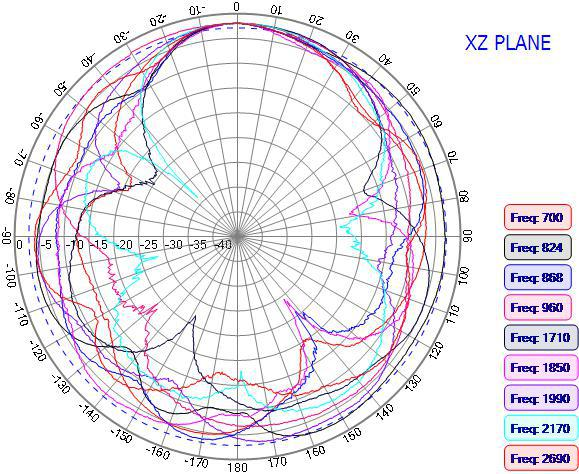## Antenna Radiation Patterns: H-Plane, E-Plane, XY XZ YZ PlanesThis article explains radiation patterns, detailing how polarization (linear and vertical) relates to the E-Plane and H-Plane, and how XY, XZ and YZ planes correspond to E-Plane and H-Plane.

A radiation pattern, or antenna pattern is a graphical representation of how a particular antenna radiates or receives energy. It is unique to an individual antenna and is made up by plotting its far field (normally radiating) radiation as charted coordinates.

You can use an antenna pattern to aid visualization of how a proposed antenna will radiate or receive electromagnetic energy when mounted. Antenna patterns are measured plotted in the orientation antenna will be used in or mounted. This is essential for planning deployment of antennas for wireless networking.

How 3D Radiation Patterns Are Represented in Two Dimensions

Antennas radiate or receive energy in all directions, making their patterns 3 dimensional. However, it is more convenient to render the pattern on separate horizontal and vertical planes known as principal plane patterns. These are essentially slices through the three dimensional field generated by the antenna.

The principal plane patterns

• The azimuth plane is also known as the XY plane and refers to the horizontal plane.
• The elevation plane is also known as the vertical or YZ plane.
• The elevation plane is oriented at right angles to the azimuth plane.
• A third perpendicular plane, the XZ plane also intersects the origin and provides data points.

These intersecting planes create complementary polar plots that provide a reliable representation of the antenna's performance. For accuracy, these plots should be derived from a true cross-section of a 3D antenna pattern, rather than plotting data in each plane.

Linearly Polarized Antennas

Polarization relates to the orientation of the electrical and magnetic components of electromagnetic waves as they are generated from a radiating antenna. In the case of linearly polarized antennas, these antennas limit the radiation of electromagnetic waves to a single linear direction. In this case, the electrical field is always in a single plane.

Depending on their orientation to the earth, antennas with linear polarization may have:

• Vertical polarization is where the electrical component of the EM wave shifts in the vertical plane.
• Horizontal polarization is where this movement is in the horizontal plane.

When interpreting the radiation patterns of linearly polarized antennas, the following planes are used.

E-PLANE (electric field plane)

E-Plane is always the plane that contains the electric component of the EM radiation and the direction of maximum radiation. The E plane will dictate whether the linear polarization is horizontal or vertical

• VERTICALLY POLARIZED antennas have an E plane that relates to the VERTICAL / YZ / ELEVATION plane.
• HORIZONTALLY POLARIZED antennas have an E plane that coincides with the HORIZONTAL / XY plane.

AZIMUTH PLANE: The Magnetic H plane (or H aperture)

This plane relates to the magnetic portion of the EM energy generated by a linearly polarized antenna. It will always be perpendicular to the E plane.

• An antenna with vertical polarization will have an H plane that coincides with the azimuth plane
• An antenna with horizontal polarization has an H plane that will coincide with the elevation plane.

radiation patterns that are labeled XY, XZ, YZ: The following applies whether the antenna is Vertically Polarized or Linearly Polarized:

• The XY radiation patterns are horizontal plane radiation patterns as they will be perpendicular to the E plane (and coincide with the H-plane of a vertically polarized antenna).
• E plane will coincide with the vertical or YZ plane. The YZ patterns are vertical plane radiation patterns.

Posted by George Hardesty on 19th Apr 2020